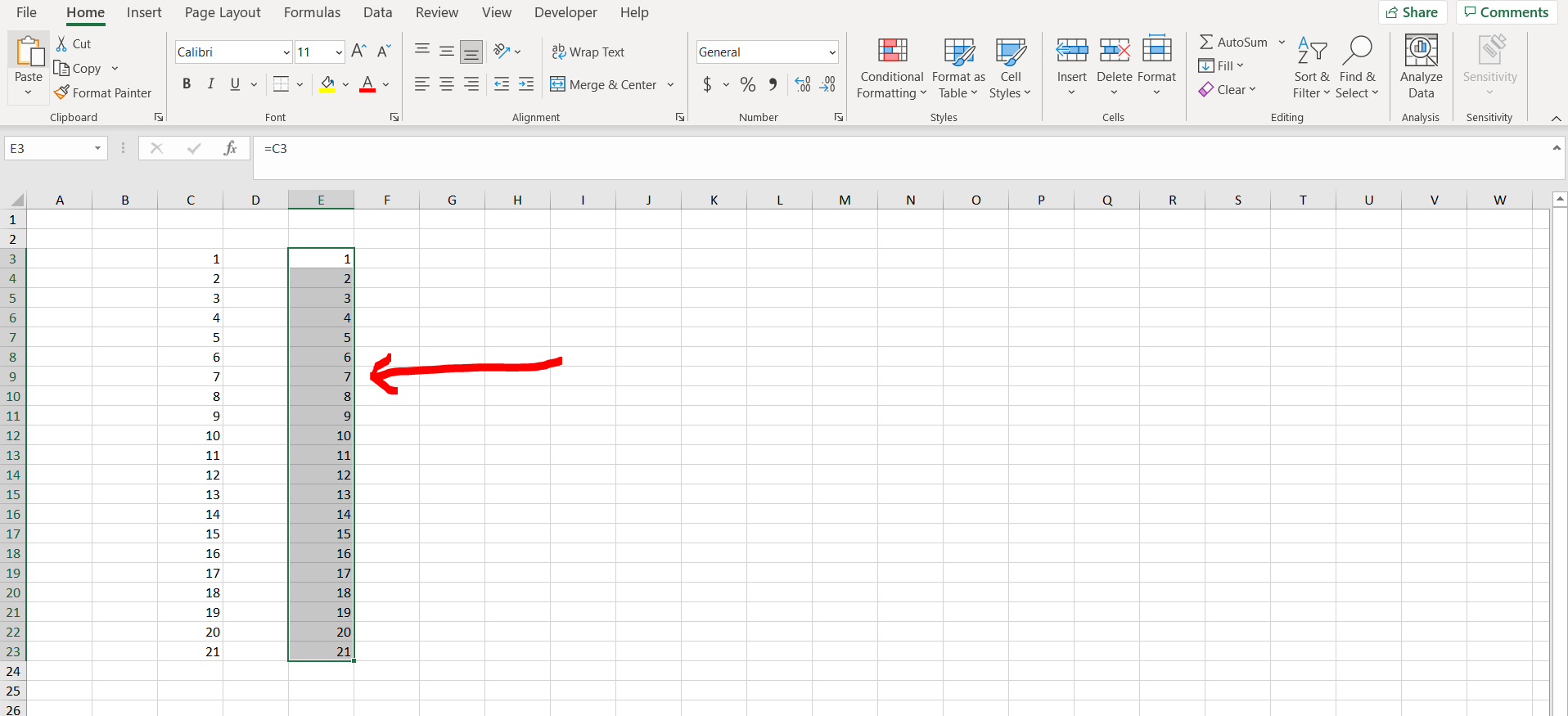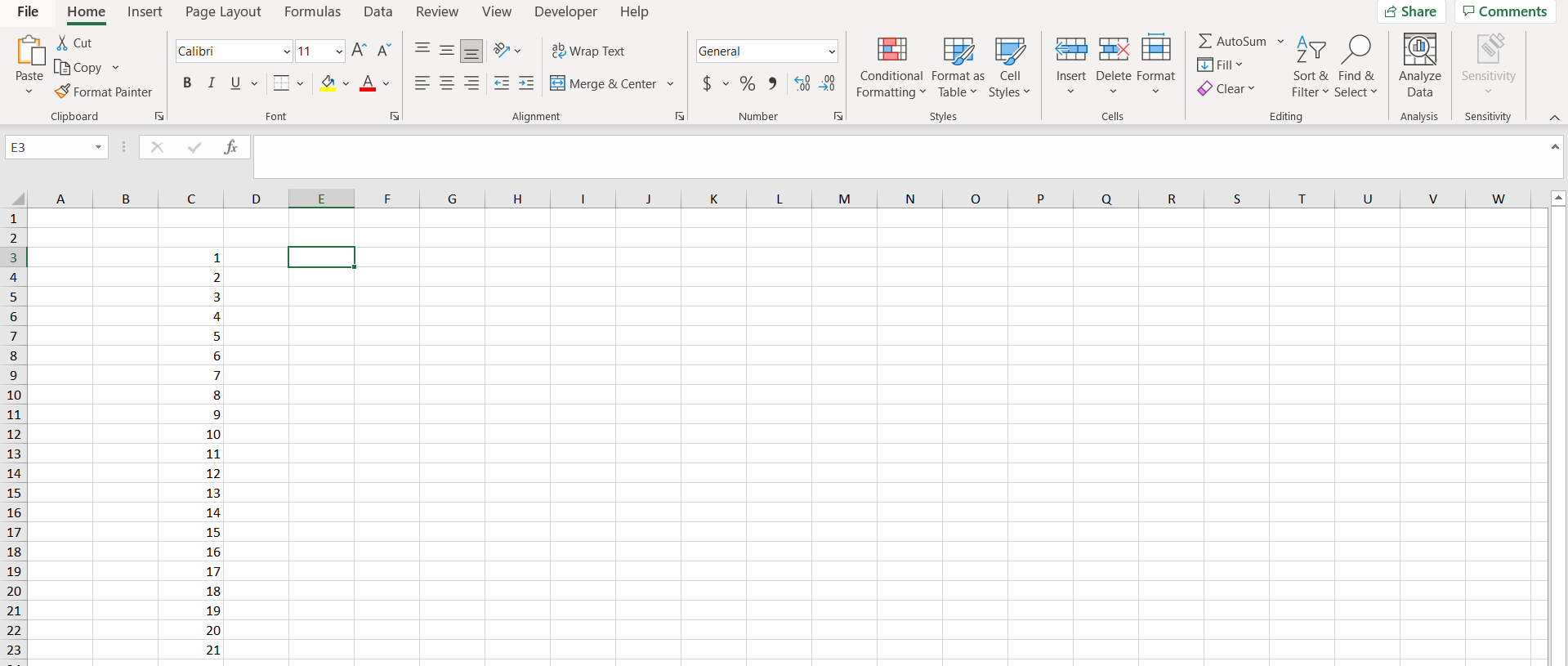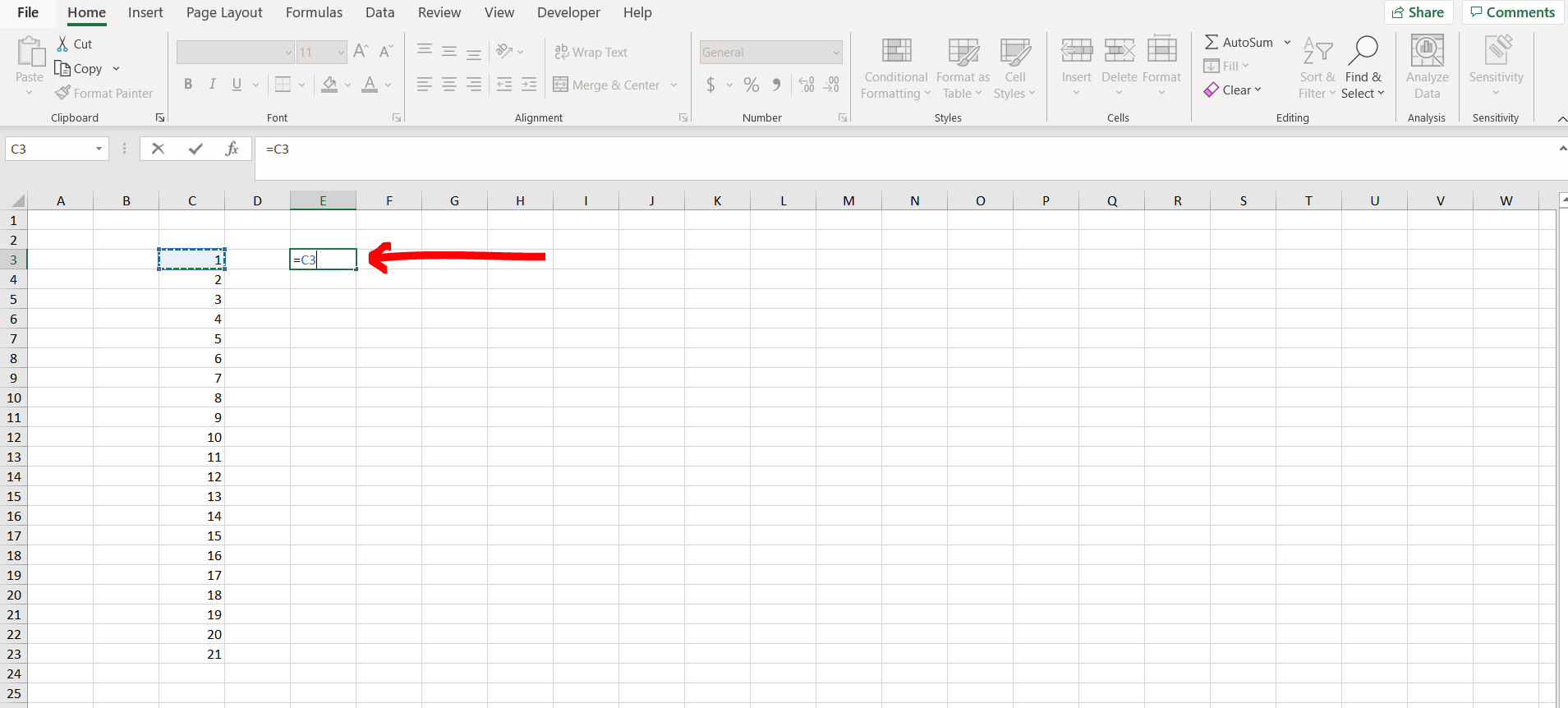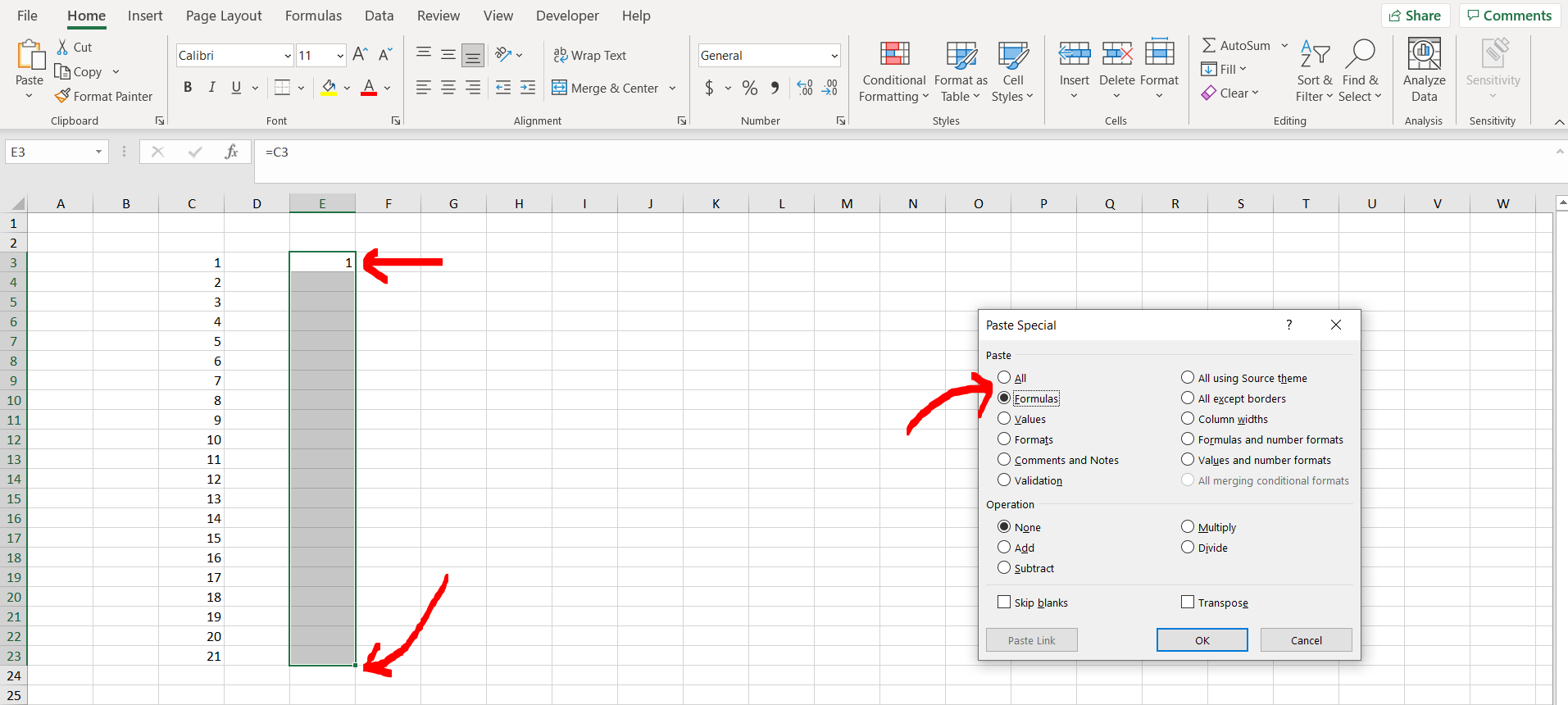# How to autofill formula in Excel without draggingExcel offers a very interesting way to autofill formula without dragging. We can cater to this problem statement by using the paste formula option. We can perform the below mentioned way to autofill formula without dragging in excel:

### Step 1 – Excel workbook with a non empty column– Open the desired Excel workbook which contains a non empty column

### Step 2 – Applying a formula– Now apply a formula to another column where you want to autofill the formula without dragging, and hit Enter.

### Step 3 – Copy the formula to entire range– Now copy the cell with formula and select the entire range of cells where you want to autofill the formula. Now press “Alt + E +S +F”. A dialogue box will appear, then hit Enter.

### Step 4 – Formula autofilled– We can see that the formula has now been autofilled till the range we selected.